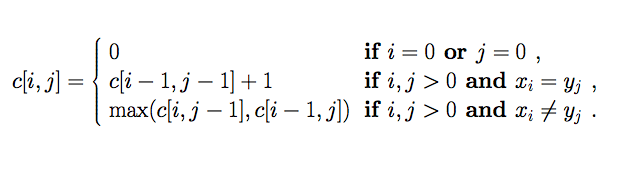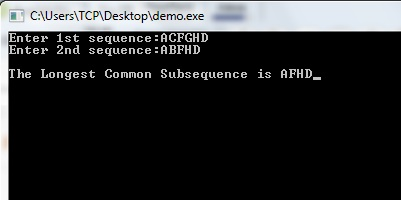# C Program for Longest Common Subsequence Problem

In this post I am sharing C program for Longest Common Subsequence Problem.
LCS problem is a dynamic programming approach in which we find the longest subsequence which is common in between two given strings. A subsequence is a sequence which appears in the same order but not necessarily contiguous. For example ACF, AFG, AFGHD, FGH are some subsequences of string ACFGHD. So for a string of length n there can be total 2^n subsequences. The LCS algorithm is widely used in bioinformatics.

For string ACFGHD and ABFHD, the longest common subsequence is AFHD. The function that is used to find the longest common subsequence of two strings is given below.Below I have shared the C program for longest common subsequence problem and a video tutorial that will help you understand LCS algorithm easily.

## C Program for Longest Common Subsequence Problem

Output### 11 thoughts on “C Program for Longest Common Subsequence Problem”

1.Thanks

2.Thanks

3.It’s very easy to understand….thanks:)

1.turedA

4.In the main() function in the scanf() statement before x and y, ‘&'(ampersand) is missing…

1.While reading string we dont have to use &.

5.Code is not giving exact answer for all the cases.
verify once again..

6.very good

7.y did u use ‘c’,’u’,’l’ n how did the compiler come to know bout it????

8.Thanks for program..this is for my open ended problem.. thanks again

9.Your logic behind this program is good bro but what if there are more then one lcs are possible. For example
I have
X = ABCBDAB
Y = BDCABA

LCS are:
BCBA
BCAB
BDAB

then how am i able to print them all ??